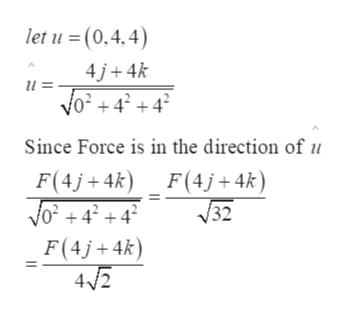# A wrench 0.6 meters long lies along the positive y-axis, and grips a bolt at the origin. A force is applied in the direction of ⟨0,4,4⟩ at the end of the wrench. Find the magnitude of the force in Newtons needed to supply 100 Newton-Meters of torque to the bolt.

Question
64 views

A wrench 0.6 meters long lies along the positive y-axis, and grips a bolt at the origin.
A force is applied in the direction of ⟨0,4,4⟩ at the end of the wrench.
Find the magnitude of the force in Newtons needed to supply 100 Newton-Meters of torque to the bolt.

check_circle

Step 1

Refer to the question, To find the toque use the formula T= r * F,  where r is the vector drawn from the bolt to the point where F is applied.

Step 2

As |r| is the length of the wrench, as the force is applied at the end of the wrench.

Step 3

We know that the wrench lies along the positive y-axis and it is given that F lies along the v...help_outlineImage Transcriptioncloselet u = (0.4,4) 4j 4k и : Vo? + 4? + 4? Since Force is in the direction of F(4j4k) F(4j+4k) 32 F(4j4k 42 fullscreen

### Want to see the full answer?

See Solution

#### Want to see this answer and more?

Solutions are written by subject experts who are available 24/7. Questions are typically answered within 1 hour.*

See Solution
*Response times may vary by subject and question.
Tagged in

### Other